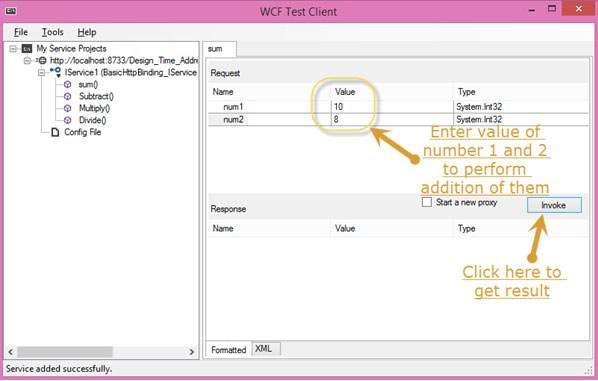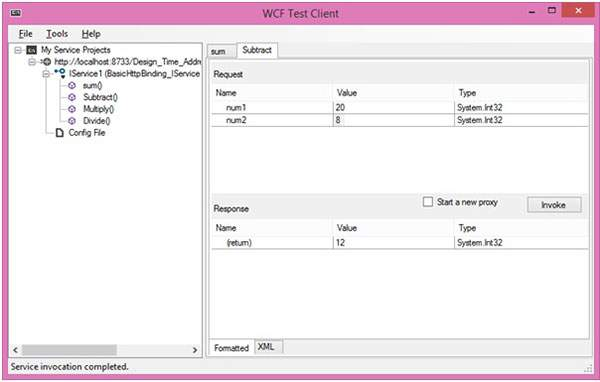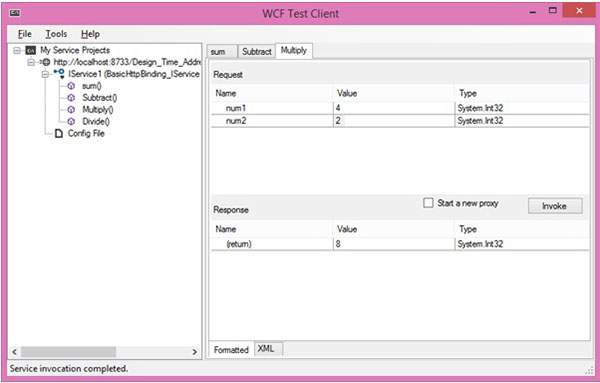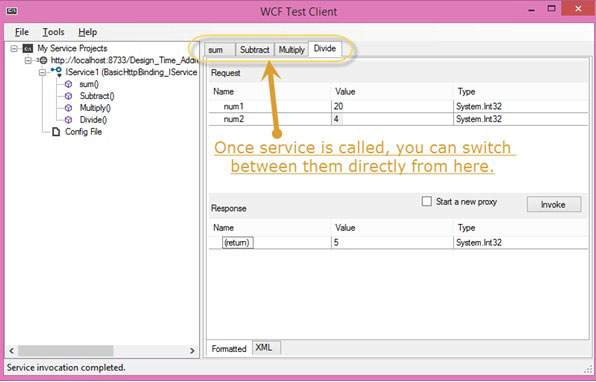# WCF - Creating WCF Service

Creating a WCF service is a simple task using Microsoft Visual Studio 2012. Given below is the step-by-step method for creating a WCF service along with all the requisite coding, to understand the concept in a better way.

• Launch Visual Studio 2012.
• Click on new project, then in Visual C# tab, select WCF option.A WCF service is created that performs basic arithmetic operations like addition, subtraction, multiplication, and division. The main code is in two different files – one interface and one class.

A WCF contains one or more interfaces and its implemented classes.

```using System;
using System.Collections.Generic;
using System.Linq;
using System.Runtime.Serialization;
using System.ServiceModel;
using System.Text;

namespace WcfServiceLibrary1 {
// NOTE: You can use the "Rename" command on the "Refactor" menu to
// change the interface name "IService1" in both code and config file
// together.

[ServiceContract]
Public interface IService1 {
[OperationContract]
int sum(int num1, int num2);

[OperationContract]
int Subtract(int num1, int num2);

[OperationContract]
int Multiply(int num1, int num2);

[OperationContract]
int Divide(int num1, int num2);
}

// Use a data contract as illustrated in the sample below to add
// composite types to service operations.

[DataContract]
Public class CompositeType {
Bool boolValue = true;
String stringValue = "Hello ";

[DataMember]
Public bool BoolValue {
get { return boolValue; }
set { boolValue = value; }
}

[DataMember]
Public string StringValue {
get { return stringValue; }
set { stringValue = value; }
}
}
}
```

The code behind its class is given below.

```using System;
usingSystem.Collections.Generic;
usingSystem.Linq;
usingSystem.Runtime.Serialization;
usingSystem.ServiceModel;
usingSystem.Text;

namespace WcfServiceLibrary1 {
// NOTE: You can use the "Rename" command on the "Refactor" menu to
// change the class name "Service1" in both code and config file
// together.

publicclassService1 :IService1 {
// This Function Returns summation of two integer numbers

publicint sum(int num1, int num2) {
return num1 + num2;
}

// This function returns subtraction of two numbers.
// If num1 is smaller than number two then this function returns 0

publicint Subtract(int num1, int num2) {
if (num1 > num2) {
return num1 - num2;
}
else {
return 0;
}
}

// This function returns multiplication of two integer numbers.
publicint Multiply(int num1, int num2) {
return num1 * num2;
}

// This function returns integer value of two integer number.
// If num2 is 0 then this function returns 1.
publicint Divide(int num1, int num2) {
if (num2 != 0) {
return (num1 / num2);
} else {
return 1;
}
}
}
}
```

To run this service, click the Start button in Visual Studio.While we run this service, the following screen appears.On clicking the sum method, the following page opens. Here, you can enter any two integer numbers and click on the Invoke button. The service will return the summation of those two numbers.Like summation, we can perform all other arithmetic operations which are listed in the menu. And here are the snaps for them.

The following page appears on clicking the Subtract method. Enter the integer numbers, click the Invoke button, and get the output as shown here −The following page appears on clicking the Multiply method. Enter the integer numbers, click the Invoke button, and get the output as shown here −The following page appears on clicking the Divide method. Enter the integer numbers, click the Invoke button, and get the output as shown here −Once the service is called, you can switch between them directly from here.